Скачать презентацию PHANTOM GRAPHS PART 2 Philip Lloyd Epsom Girls

c9a0866175e49455fa2c737fd1c2596d.ppt

• Количество слайдов: 41PHANTOM GRAPHS PART 2 Philip Lloyd Epsom Girls Grammar School Web site: www. phantomgraphs. weebly. com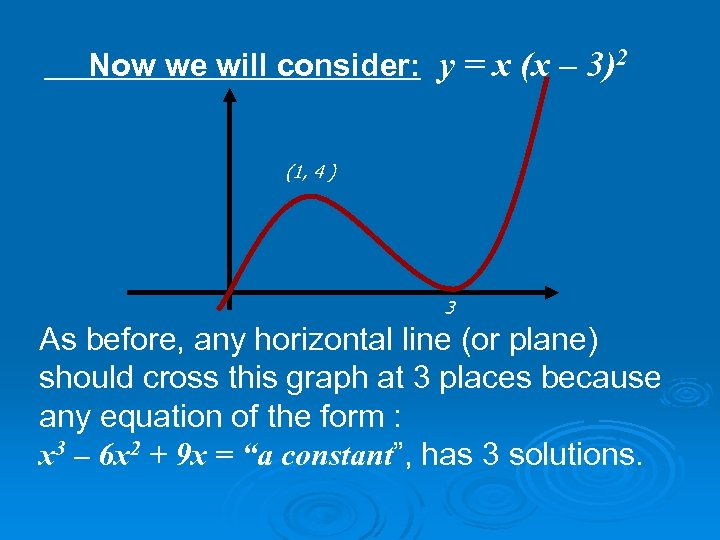Now we will consider: y = x (x – 3)2 (1, 4 ) 3 As before, any horizontal line (or plane) should cross this graph at 3 places because any equation of the form : x 3 – 6 x 2 + 9 x = “a constant”, has 3 solutions.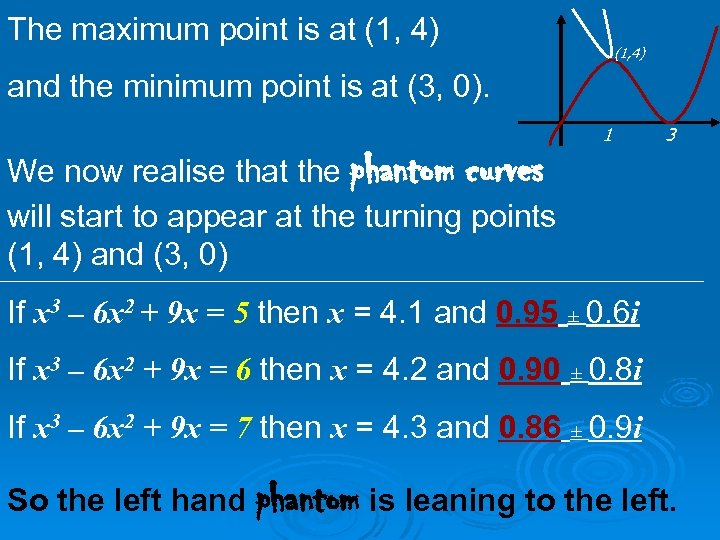The maximum point is at (1, 4) and the minimum point is at (3, 0). We now realise that the phantom curves will start to appear at the turning points (1, 4) and (3, 0) 1 3 If x 3 – 6 x 2 + 9 x = 5 then x = 4. 1 and 0. 95 ± 0. 6 i If x 3 – 6 x 2 + 9 x = 6 then x = 4. 2 and 0. 90 ± 0. 8 i If x 3 – 6 x 2 + 9 x = 7 then x = 4. 3 and 0. 86 ± 0. 9 i So the left hand phantom is leaning to the left.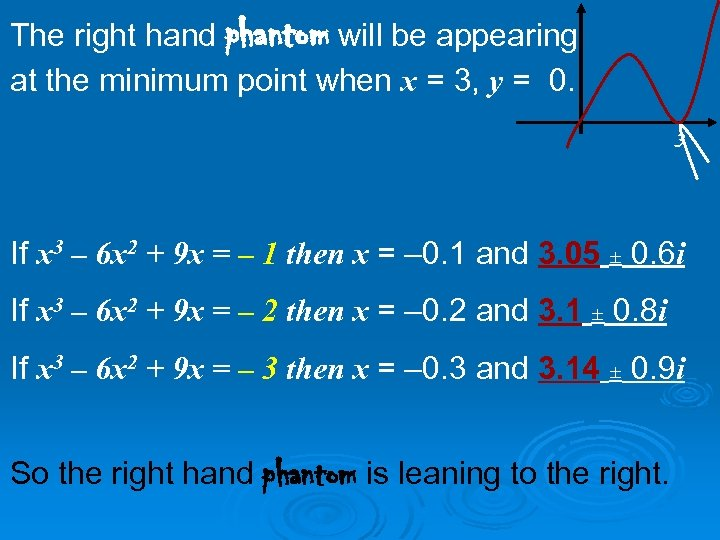The right hand phantom will be appearing at the minimum point when x = 3, y = 0. 3 If x 3 – 6 x 2 + 9 x = – 1 then x = – 0. 1 and 3. 05 ± 0. 6 i If x 3 – 6 x 2 + 9 x = – 2 then x = – 0. 2 and 3. 1 ± 0. 8 i If x 3 – 6 x 2 + 9 x = – 3 then x = – 0. 3 and 3. 14 ± 0. 9 i So the right hand phantom is leaning to the right.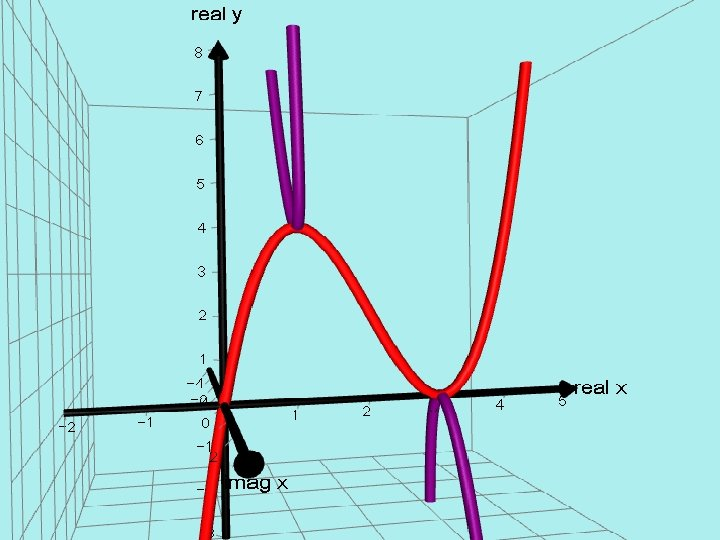AUTOGRAPH VERSION. = x(x - 3)²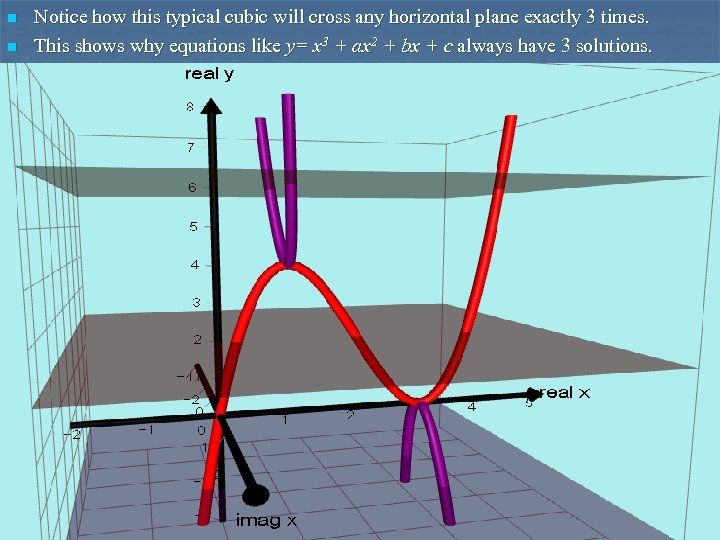n n Notice how this typical cubic will cross any horizontal plane exactly 3 times. This shows why equations like y= x 3 + ax 2 + bx + c always have 3 solutions.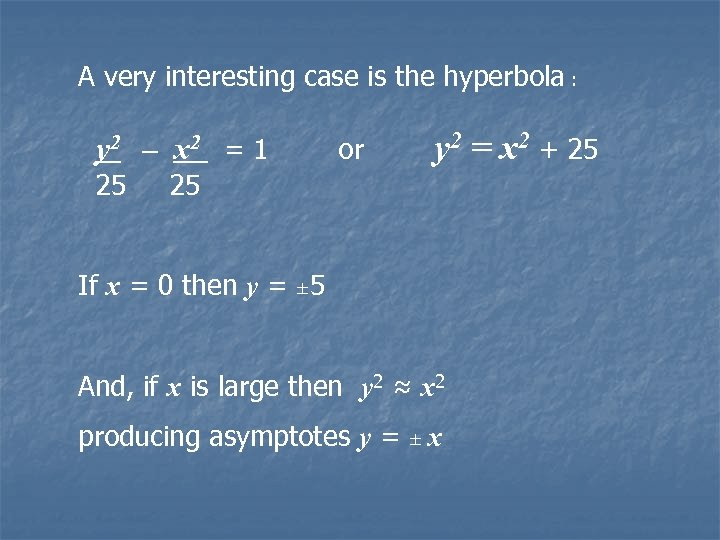A very interesting case is the hyperbola : y 2 – x 2 = 1 25 or y 2 = x 2 + 25 25 If x = 0 then y = ± 5 And, if x is large then y 2 ≈ x 2 producing asymptotes y = ± x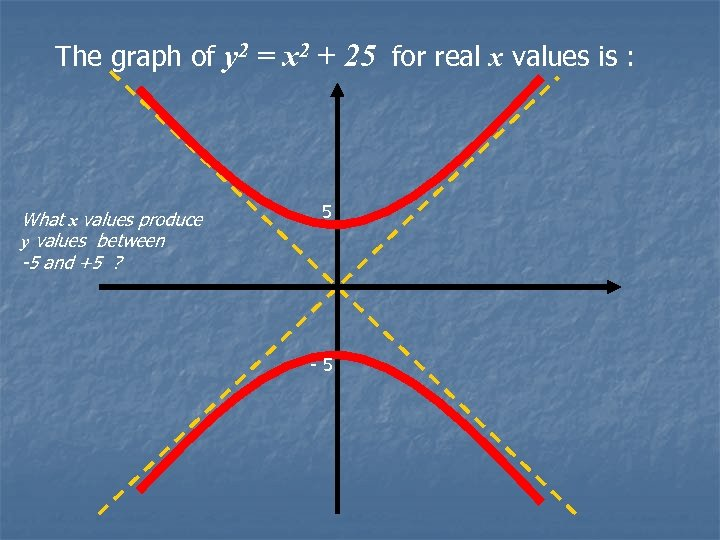The graph of y 2 = x 2 + 25 for real x values is : What x values produce y values between -5 and +5 ? 5 -5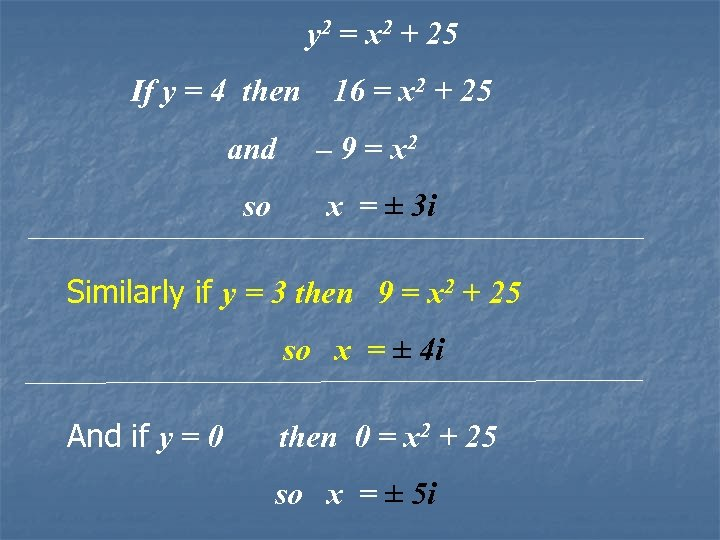y 2 = x 2 + 25 If y = 4 then 16 = x 2 + 25 and – 9 = x 2 so x = ± 3 i Similarly if y = 3 then 9 = x 2 + 25 so x = ± 4 i And if y = 0 then 0 = x 2 + 25 so x = ± 5 i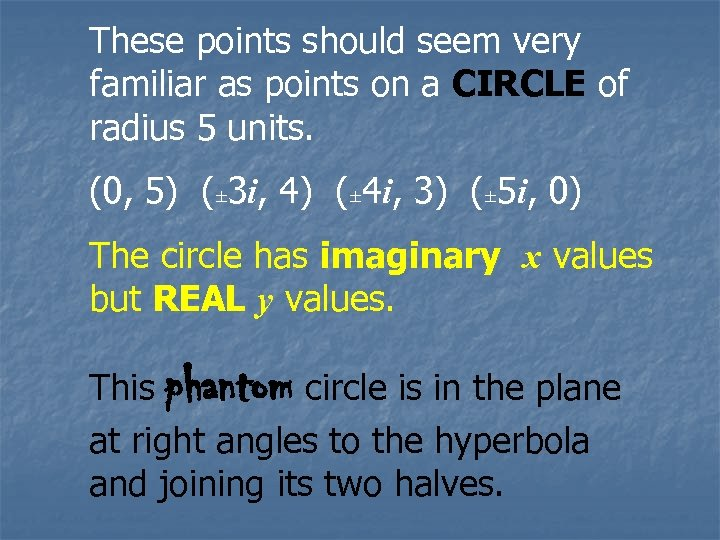These points should seem very familiar as points on a CIRCLE of radius 5 units. (0, 5) (± 3 i, 4) (± 4 i, 3) (± 5 i, 0) The circle has imaginary x values but REAL y values. This phantom circle is in the plane at right angles to the hyperbola and joining its two halves.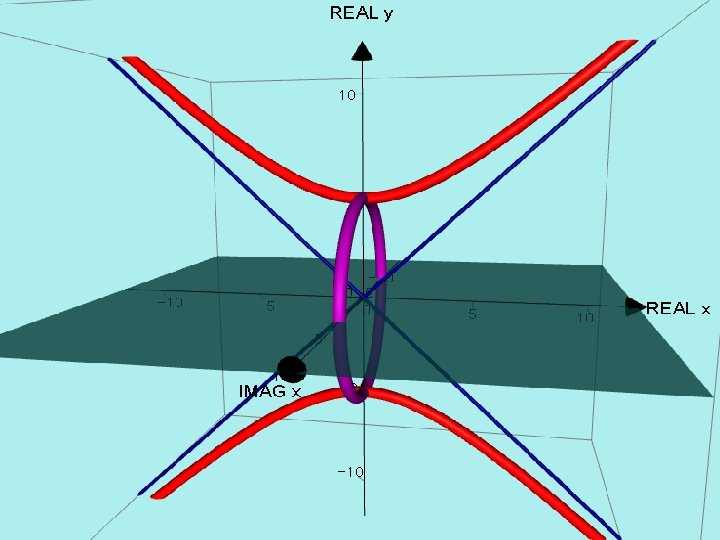AUTOGRAPH VERSION. y² = x² + 25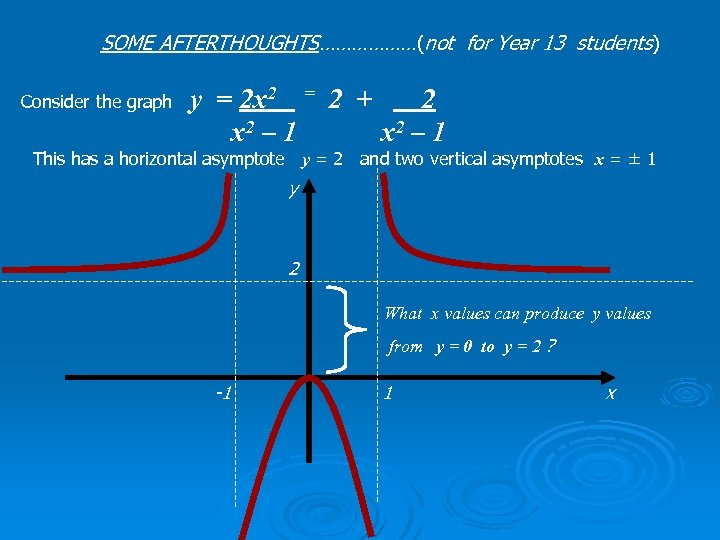SOME AFTERTHOUGHTS………………(not for Year 13 students) y = 2 x 2 = 2 + 2 x 2 – 1 Consider the graph This has a horizontal asymptote y = 2 and two vertical asymptotes x = ± 1 y 2 What x values can produce y values from y = 0 to y = 2 ? -1 1 x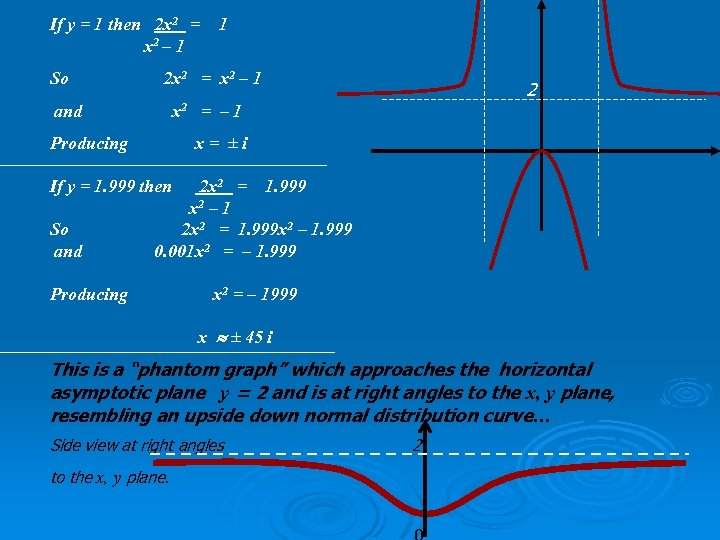If y = 1 then 2 x 2 = 1 x 2 – 1 So 2 x 2 = x 2 – 1 2 and x 2 = – 1 Producing x = ± i If y = 1. 999 then 2 x 2 = 1. 999 x 2 – 1 So 2 x 2 = 1. 999 x 2 – 1. 999 and 0. 001 x 2 = – 1. 999 Producing x 2 = – 1999 x ± 45 i This is a “phantom graph” which approaches the horizontal asymptotic plane y = 2 and is at right angles to the x, y plane, resembling an upside down normal distribution curve… Side view at right angles 2 to the x, y plane.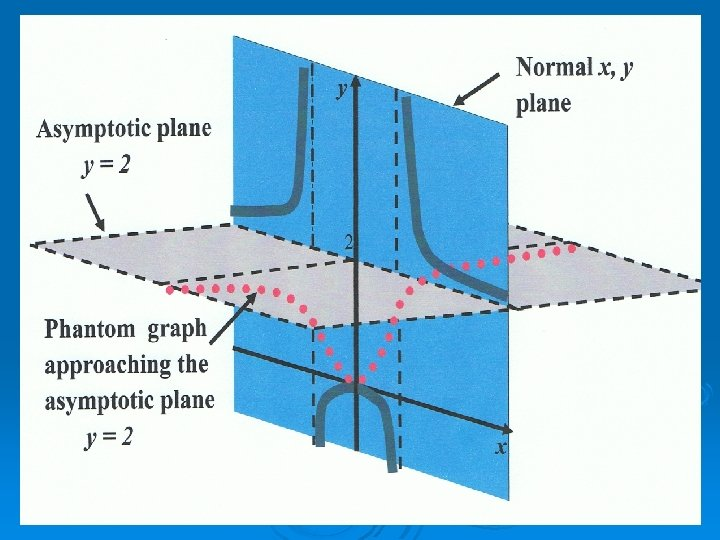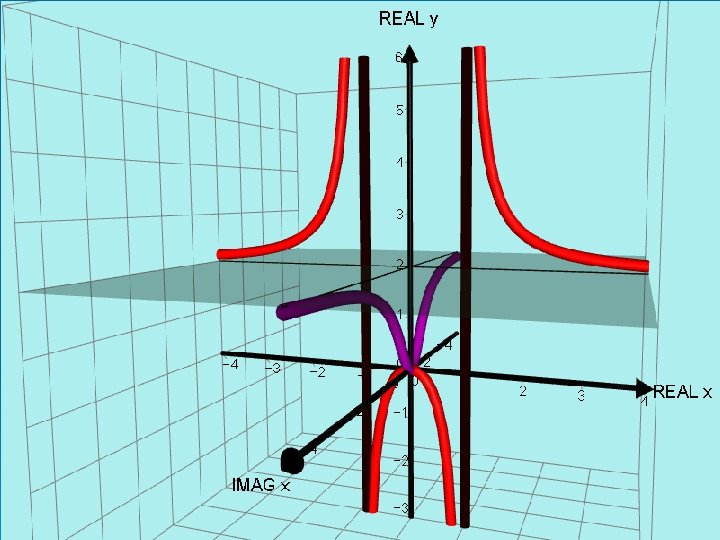AUTOGRAPH VERSION. Asymptotic plane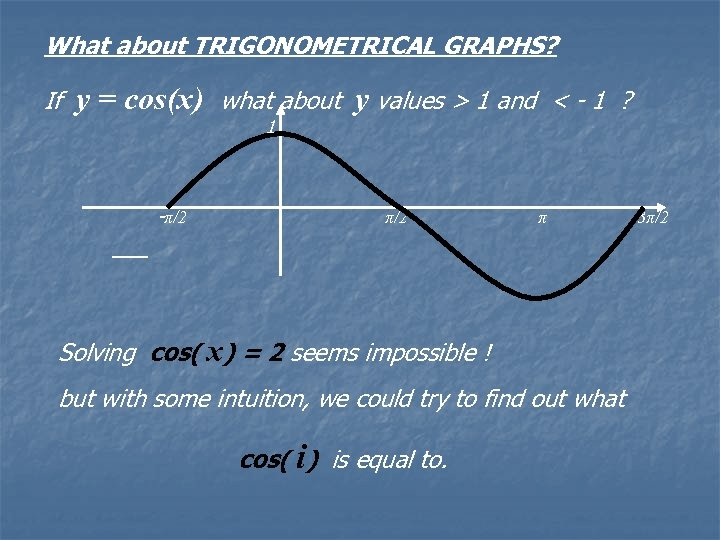What about TRIGONOMETRICAL GRAPHS? If y = cos(x) what about y values > 1 and < - 1 ? 1 -π/2 π Solving cos( x) = 2 seems impossible ! but with some intuition, we could try to find out what cos( i) is equal to. 3π/2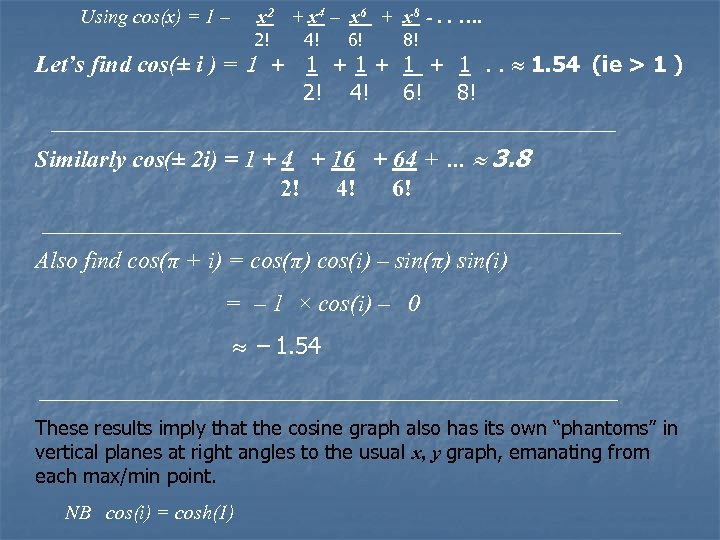Using cos(x) = 1 – x 2 + x 4 – x 6 + x 8 -. . …. 2! 4! 6! 8! Let’s find cos(± i ) = 1 + 1 + 1. 54 (ie > 1 ) 2! 4! 6! 8! Similarly cos(± 2 i) = 1 + 4 + 16 + 64 + … 3. 8 2! 4! 6! Also find cos(π + i) = cos(π) cos(i) – sin(π) sin(i) = – 1 × cos(i) – 0 – 1. 54 These results imply that the cosine graph also has its own “phantoms” in vertical planes at right angles to the usual x, y graph, emanating from each max/min point. NB cos(i) = cosh(1)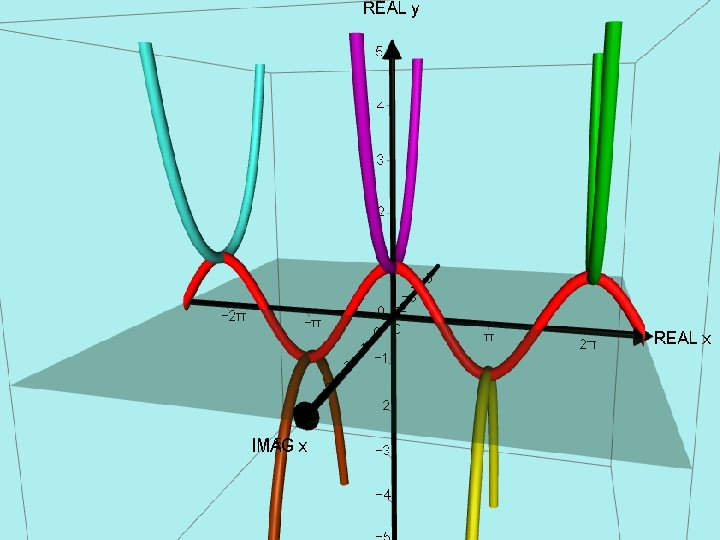-π/2 π 3π/2 2π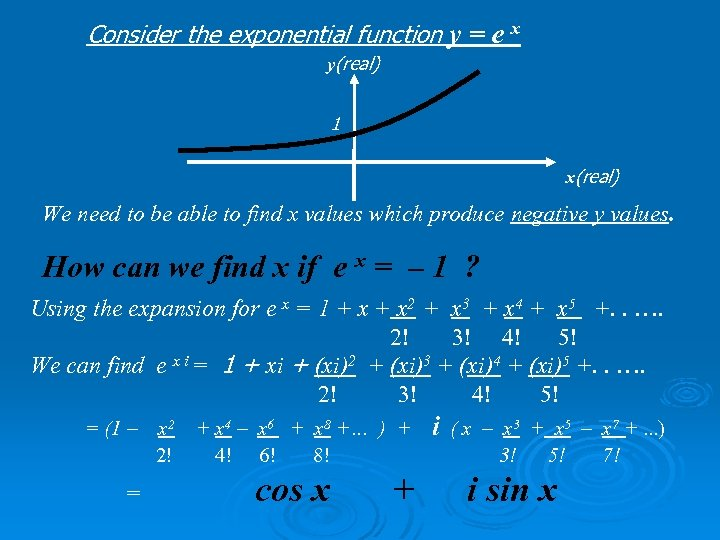Consider the exponential function y = e x y(real) 1 x(real) We need to be able to find x values which produce negative y values. How can we find x if e x = – 1 ? Using the expansion for e x = 1 + x 2 + x 3 + x 4 + x 5 +. . …. 2! 3! 4! 5! We can find e x i = 1 + xi + (xi)2 + (xi)3 + (xi)4 + (xi)5 +. . …. 2! 3! 4! 5! = (1 – = x 2 + x 4 – x 6 + x 8 +… ) + 2! 4! 6! 8! i ( x – x 3 + x 5 – x 7 +. . . ) 3! 5! 7! cos x + i sin x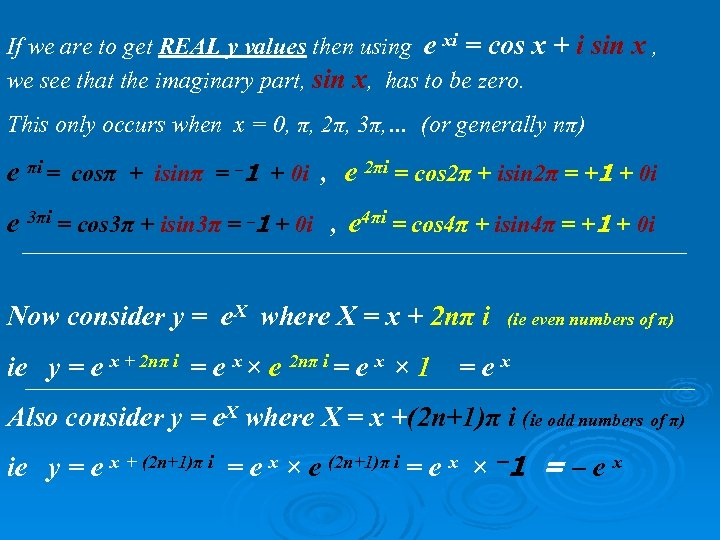If we are to get REAL y values then using e xi = cos x + i sin x , we see that the imaginary part, sin x, has to be zero. This only occurs when x = 0, π, 2π, 3π, … (or generally nπ) e πi = cosπ + isinπ = – 1 + 0 i , e 2πi = cos 2π + isin 2π = +1 + 0 i e 3πi = cos 3π + isin 3π = – 1 + 0 i , e 4πi = cos 4π + isin 4π = +1 + 0 i Now consider y = e. X where X = x + 2 nπ i (ie even numbers of π) ie y = e x + 2 nπ i = e x × e 2 nπ i = e x × 1 = e x Also consider y = e. X where X = x +(2 n+1)π i (ie odd numbers of π) ie y = e x + (2 n+1)π i = e x × e (2 n+1)π i = e x × – 1 = – e x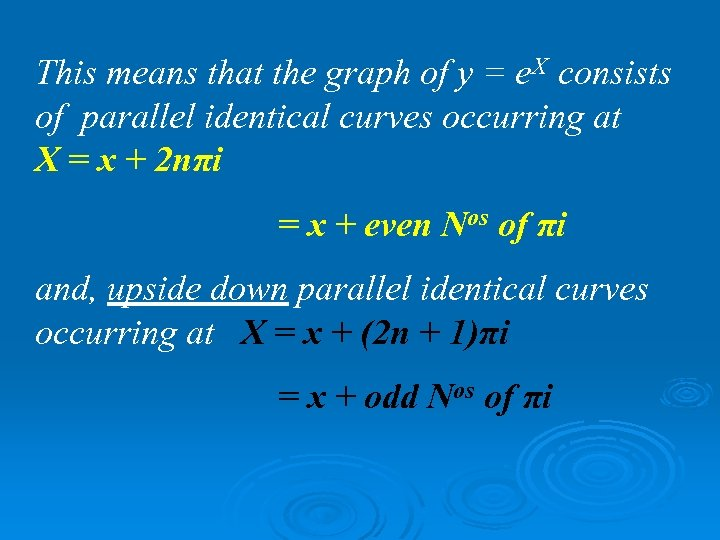This means that the graph of y = e. X consists of parallel identical curves occurring at X = x + 2 nπi = x + even Nos of πi and, upside down parallel identical curves occurring at X = x + (2 n + 1)πi = x + odd Nos of πi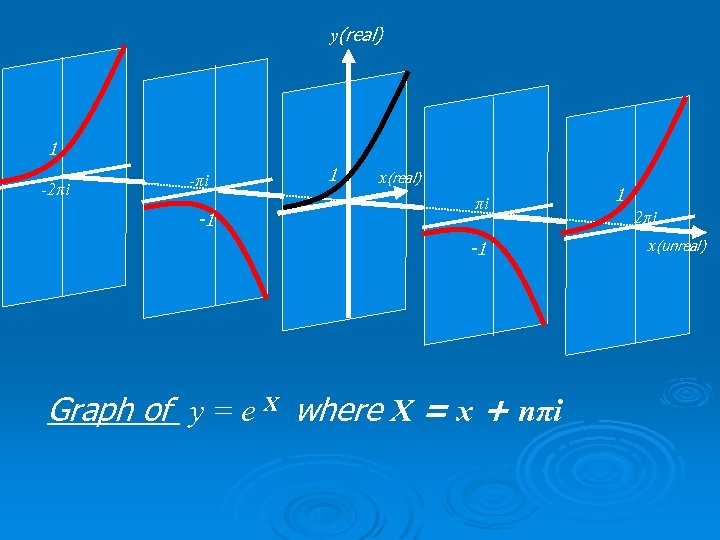y(real) 1 -2πi -1 1 x(real) πi -1 Graph of y = e X where X = x + nπi 1 2πi x(unreal)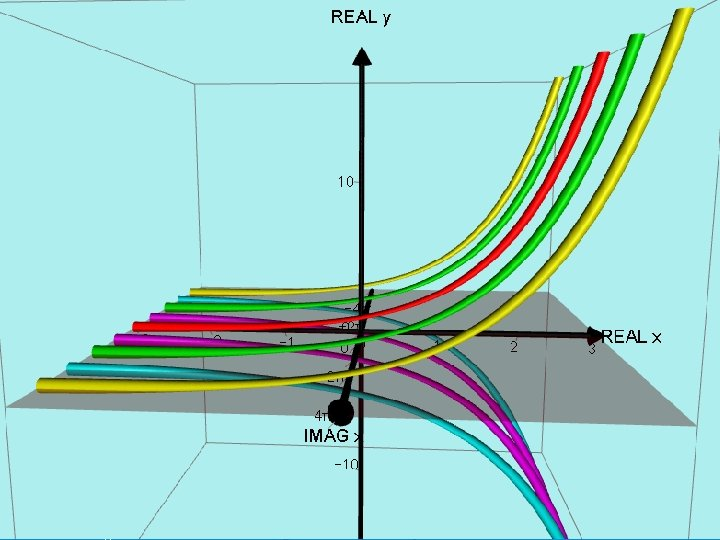Graph of y = e X where X = x + nπi x(unreal) y(real) 2π π -π x(real) - 2π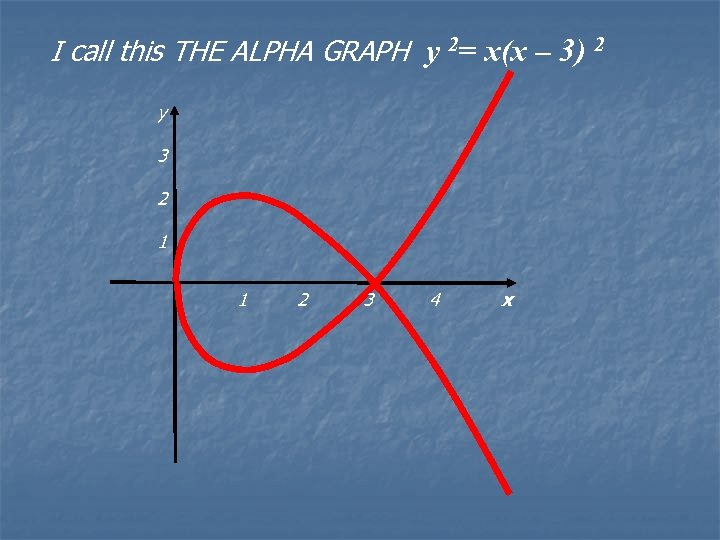I call this THE ALPHA GRAPH y 2= x(x – 3) 2 y 3 2 1 1 2 3 4 x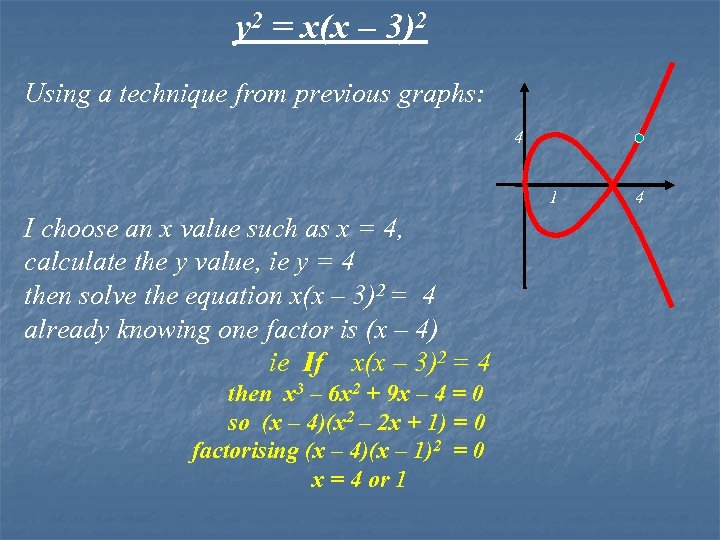y 2 = x(x – 3)2 Using a technique from previous graphs: 4 1 I choose an x value such as x = 4, calculate the y value, ie y = 4 then solve the equation x(x – 3)2 = 4 already knowing one factor is (x – 4) ie If x(x – 3)2 = 4 then x 3 – 6 x 2 + 9 x – 4 = 0 so (x – 4)(x 2 – 2 x + 1) = 0 factorising (x – 4)(x – 1)2 = 0 x = 4 or 1 4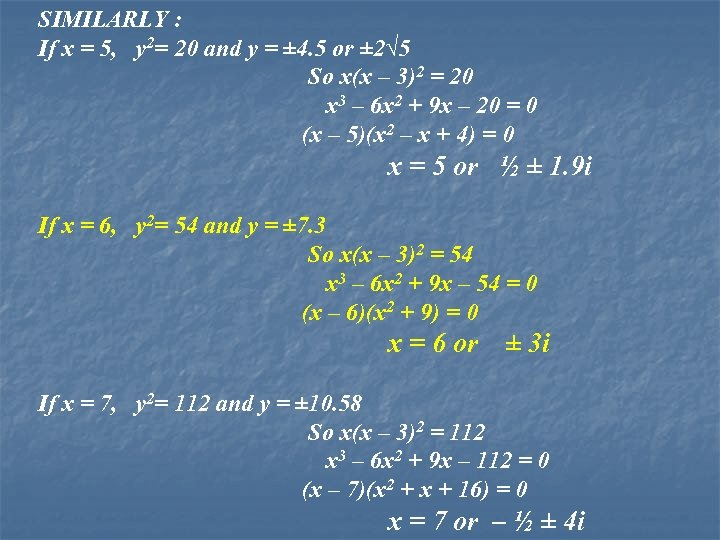SIMILARLY : If x = 5, y 2= 20 and y = ± 4. 5 or ± 2√ 5 So x(x – 3)2 = 20 x 3 – 6 x 2 + 9 x – 20 = 0 (x – 5)(x 2 – x + 4) = 0 x = 5 or ½ ± 1. 9 i If x = 6, y 2= 54 and y = ± 7. 3 So x(x – 3)2 = 54 x 3 – 6 x 2 + 9 x – 54 = 0 (x – 6)(x 2 + 9) = 0 x = 6 or ± 3 i If x = 7, y 2= 112 and y = ± 10. 58 So x(x – 3)2 = 112 x 3 – 6 x 2 + 9 x – 112 = 0 (x – 7)(x 2 + x + 16) = 0 x = 7 or – ½ ± 4 i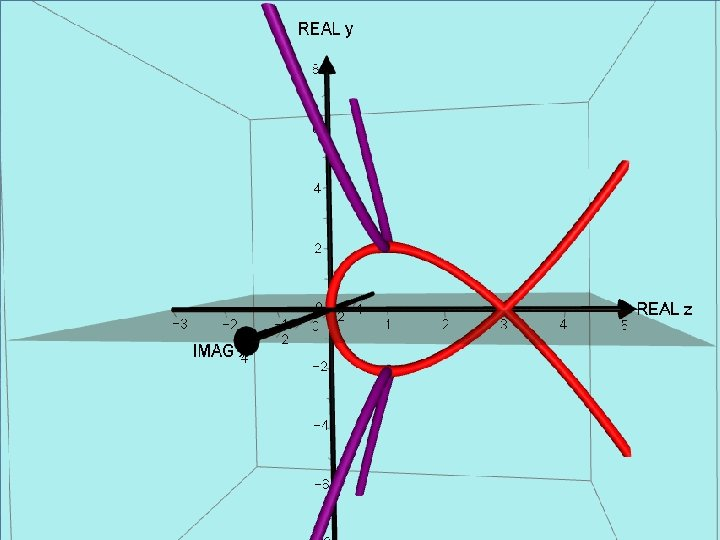AUTOGRAPH VERSION. autographmaths. com/activities/ philiplloyd/loading. ht ml? activity=009 phantom. html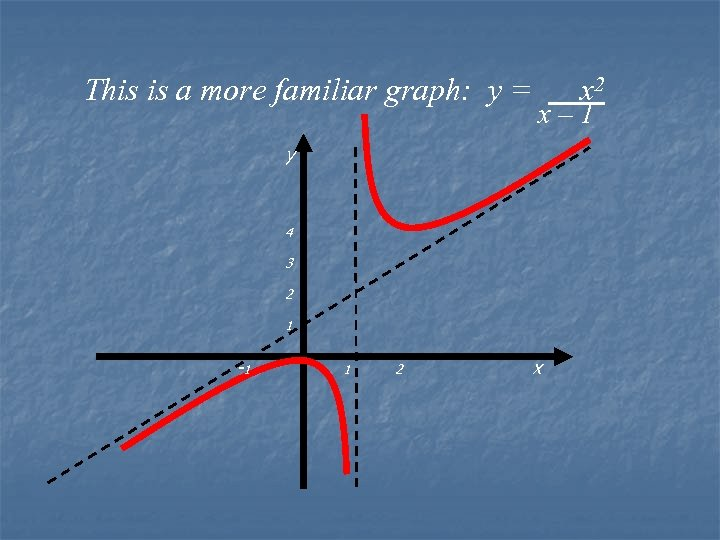This is a more familiar graph: y = x 2 x– 1 y 4 3 2 1 -1 1 2 x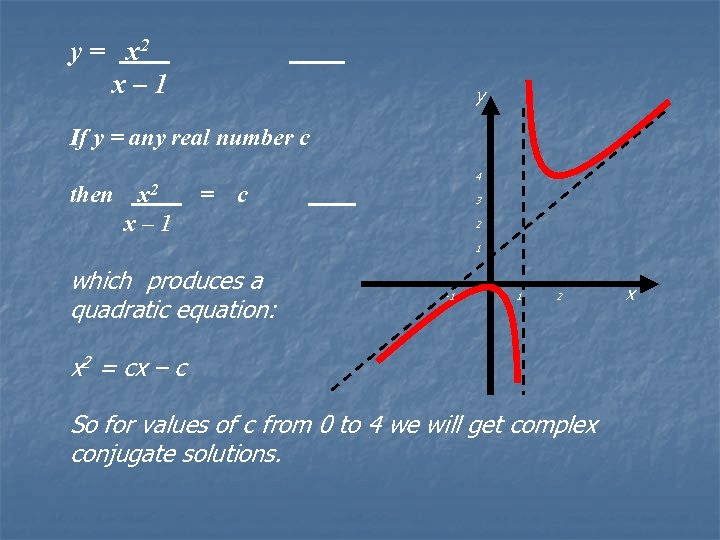y = x 2 x – 1 y If y = any real number c then x 2 = c x – 1 4 3 2 1 which produces a quadratic equation: x -1 1 2 x 2 = cx – c So for values of c from 0 to 4 we will get complex conjugate solutions.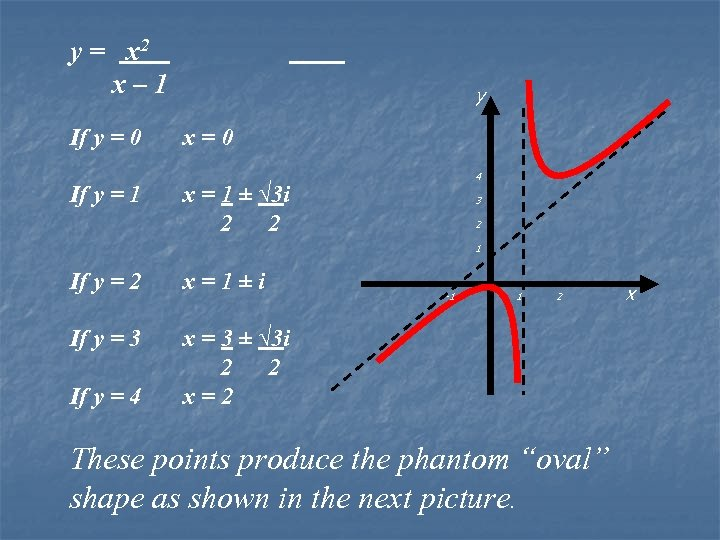y = x 2 x – 1 If y = 0 x = 0 If y = 1 x = 1 ± √ 3 i 2 2 If y = 2 x = 1 ± i If y = 3 x = 3 ± √ 3 i 2 2 If y = 4 x = 2 y 4 3 2 1 x -1 1 2 These points produce the phantom “oval” shape as shown in the next picture.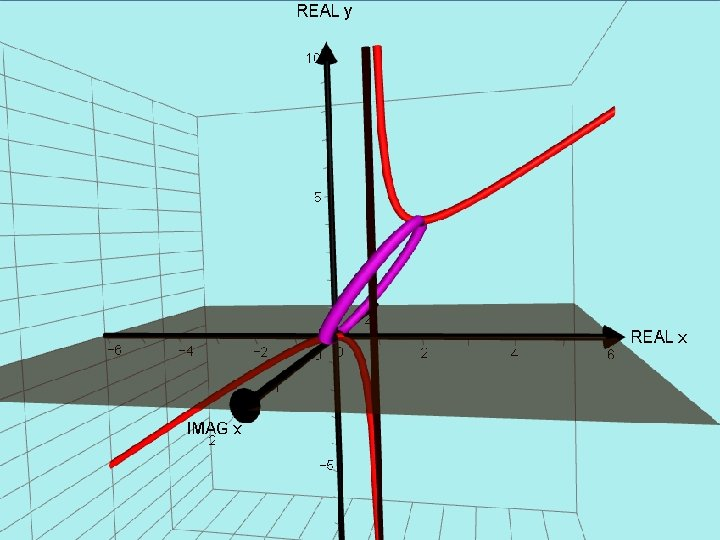AUTOGRAPH VERSION. y = x²/(x - 1)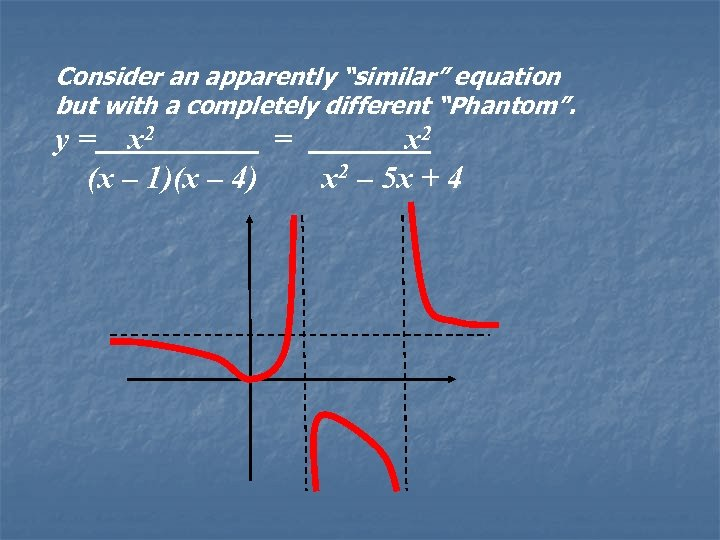Consider an apparently “similar” equation but with a completely different “Phantom”. y = x 2 = x 2 (x – 1)(x – 4) x 2 – 5 x + 4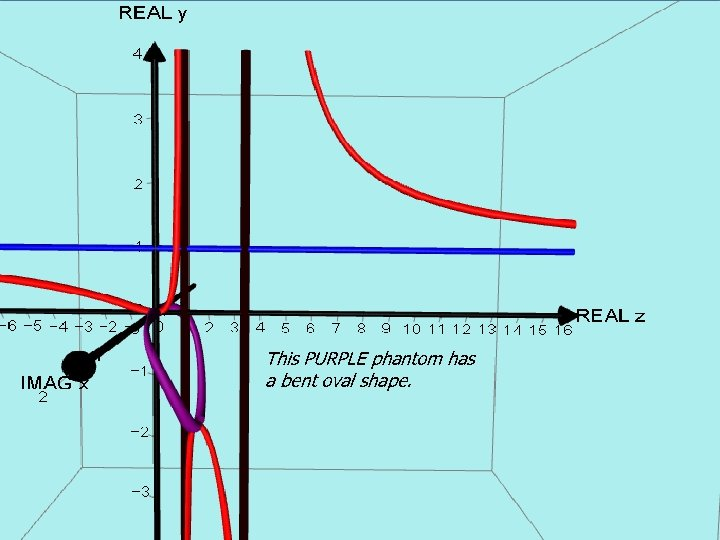AUTOGRAPH VERSION. Curve with 2 vertical asymptotes This PURPLE phantom has a bent oval shape.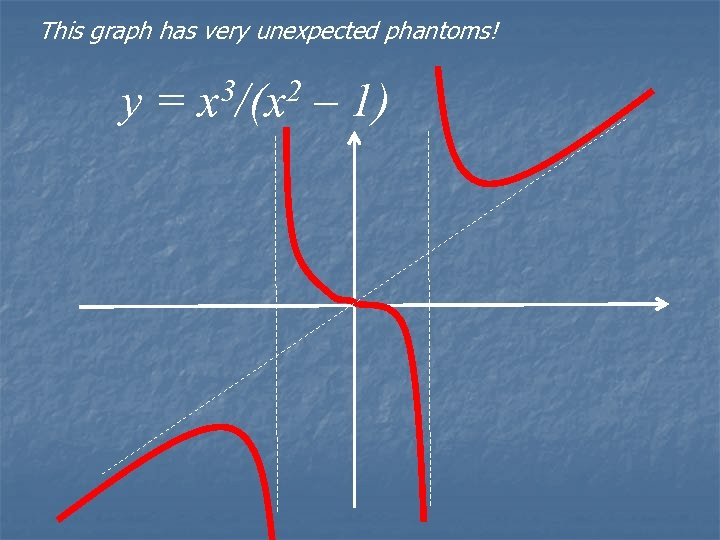This graph has very unexpected phantoms! y = x 3/(x 2 – 1)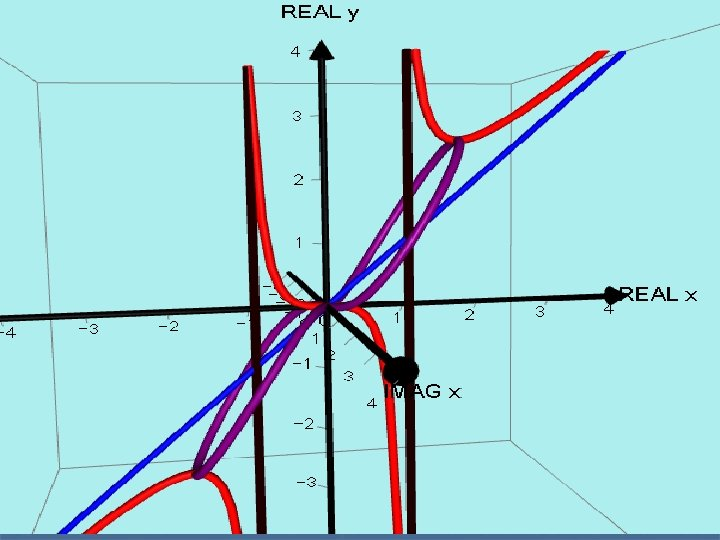AUTOGRAPH VERSION. y = x³/(x² - 1)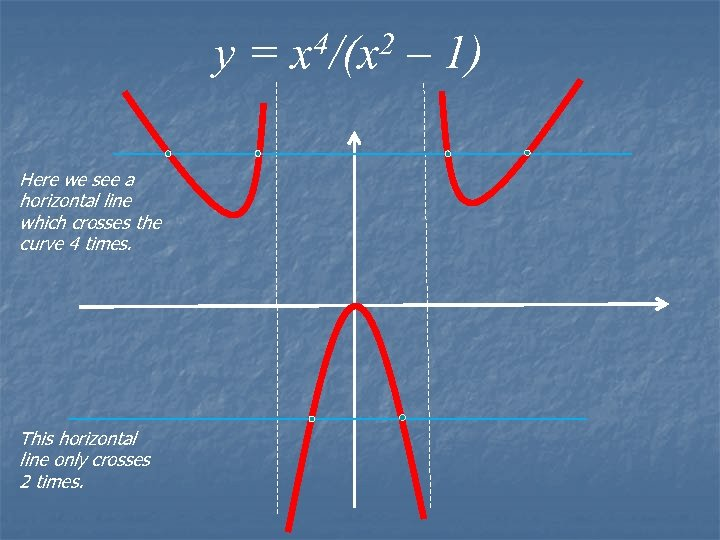y = x 4/(x 2 – 1) Here we see a horizontal line which crosses the curve 4 times. This horizontal line only crosses 2 times.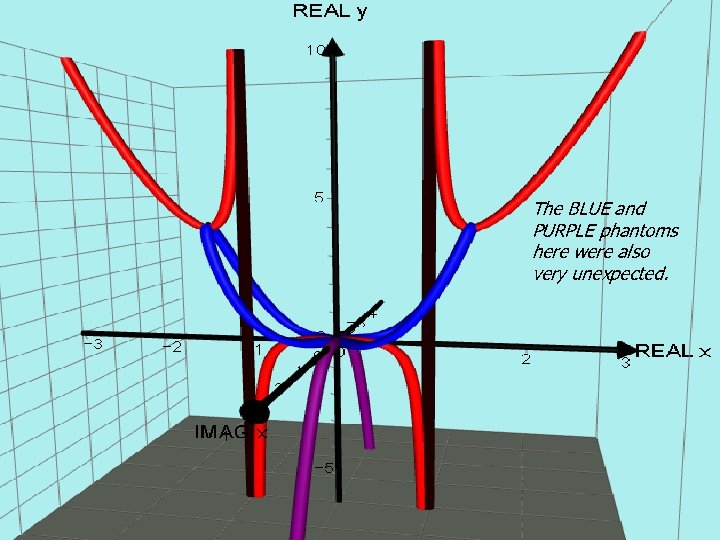AUTOGRAPH VERSION. y = x^4/(x² - 1) The BLUE and PURPLE phantoms here were also very unexpected.THE END Please check out the Web site: www. phantomgraphs. weebly. com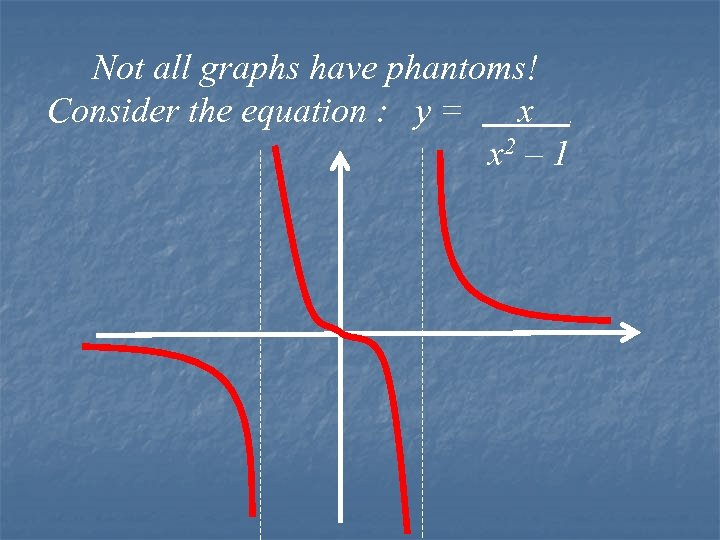Not all graphs have phantoms! Consider the equation : y = x x 2 – 1 .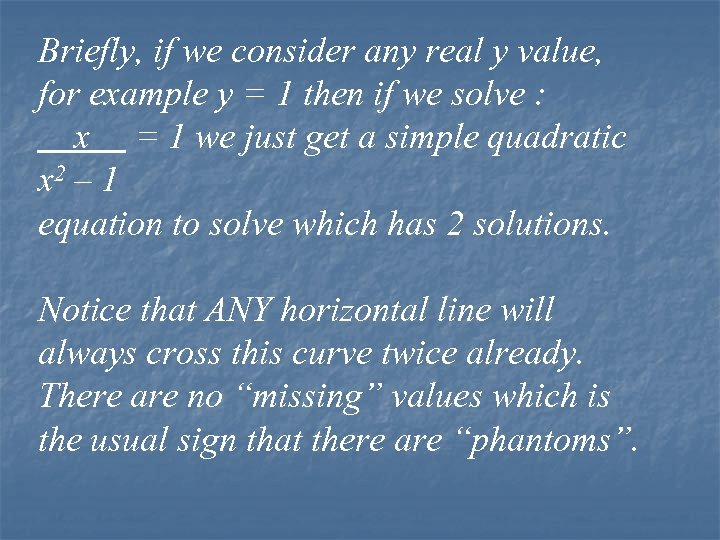Briefly, if we consider any real y value, for example y = 1 then if we solve : x = 1 we just get a simple quadratic x 2 – 1 equation to solve which has 2 solutions. Notice that ANY horizontal line will always cross this curve twice already. There are no “missing” values which is the usual sign that there are “phantoms”.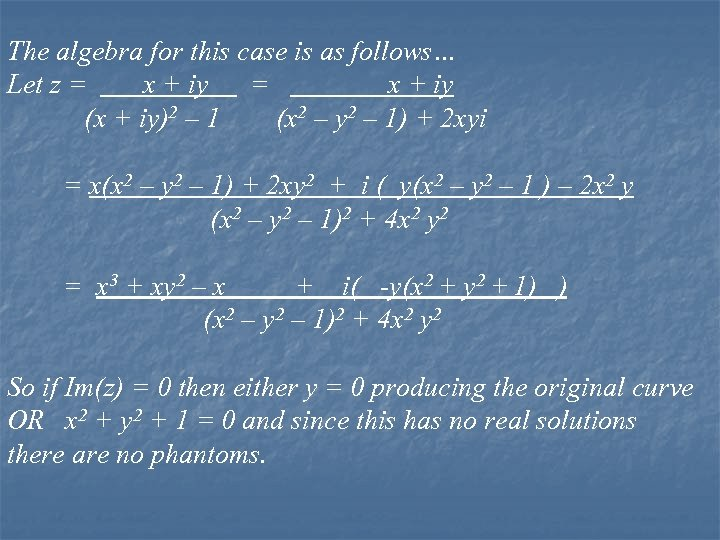The algebra for this case is as follows… Let z = x + iy (x + iy)2 – 1 (x 2 – y 2 – 1) + 2 xyi = x(x 2 – y 2 – 1) + 2 xy 2 + i ( y(x 2 – y 2 – 1 ) – 2 x 2 y (x 2 – y 2 – 1)2 + 4 x 2 y 2 = x 3 + xy 2 – x + i( -y(x 2 + y 2 + 1) ) (x 2 – y 2 – 1)2 + 4 x 2 y 2 So if Im(z) = 0 then either y = 0 producing the original curve OR x 2 + y 2 + 1 = 0 and since this has no real solutions there are no phantoms.